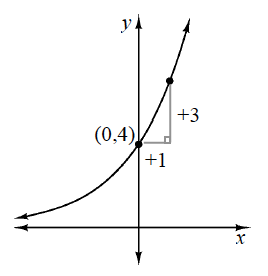### Home > GB8I > Chapter 8 Unit 9 > Lesson INT1: 8.1.5 > Problem8-70

8-70.

Use the clues in the graph below to write a possible corresponding equation in $y = ab^{x}$ form.Use the information on the graph to find the coordinates of the unlabeled point.

The two points on the graph are $(0,4)$ and $(1,7)$.

What is the multiplier, or common ratio?

$y = 4(1.75)^x$